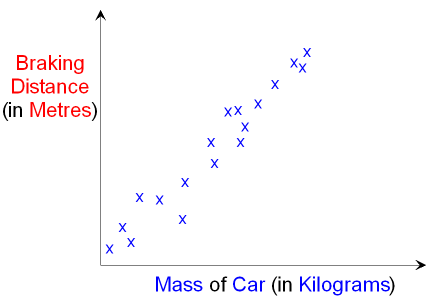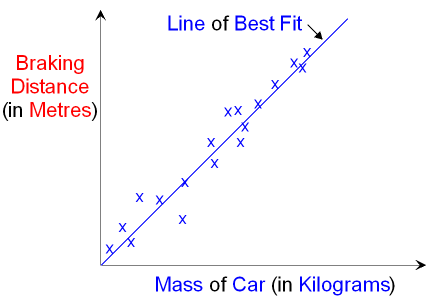gcsescience.com                                       16                                       gcsescience.com

How Science Works

How to Present Data - Line Graph - Scattergram.

There are a number of different ways of presenting data.

When both the independent variable and the dependent variable
are continuous, then the data is best shown as a line graph.

A graph showing the individual points of one variable
plotted against the other variable is called a scattergram.

For example, the graph below shows a scattergram
of the mass of a car plotted against its braking distance.A line of best fit can show the relationship between the variables.
In this case the relationship is directly proportional.Other forms of data can be presented as a bar chart.

gcsescience.com         Chemistry Index         Physics Index         gcsescience.com